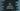# 3 ways to find the exponent without using pow() method in C++## How to find the exponent without using pow() method in C++:

We can find the exponent without using the pow() method in C++. We can use a loop or a recursive method. To find a^b, we have to multiply a with itself for b number of times.

In this post, we will learn how to calculate the exponent by using a for loop, while loop and with a recursive method.

### Example 1: C++ program find the exponent by using for loops:

The below program uses for loops to find the exponent value. It takes two numbers as inputs from the user and finds the exponent using a for loop.

``````#include <iostream>
using namespace std;

long power(long b, long e)
{
long p = 1;

for (int i = 0; i < e; i++)
{
p *= b;
}

return p;
}

int main()
{
long b, e;

cout << "Enter the base: " << endl;
cin >> b;

cout << "Enter the exponent: " << endl;
cin >> e;

cout << b << "^" << e << "=" << power(b, e) << endl;
}``````

In this example,

• The base and exponent values are stored in b and e variables. Both are long variables.
• The power method takes the base and exponent values as its arguments.

• It uses a for loop to find the power. Variable p is used to hold the power. It is initialized as 1.
• The for loop runs for e number of times and on each iteration, it multiplies current value of p with b and save that value in p.
• Once the loop ends, p will hold the value of the required exponent.

It will give output as like below:

``````Enter the base:
3
Enter the exponent:
4
3^4=81

Enter the base:
22
Enter the exponent:
0
22^0=1``````

### Example 2: C++ program find the exponent by using while loops:

We can also write the above program using while loops. It will work similarly.

``````#include <iostream>
using namespace std;

long power(long b, long e)
{
long p = 1;

while(e--){
p *= b;
}

return p;
}

int main()
{
long b, e;

cout << "Enter the base: " << endl;
cin >> b;

cout << "Enter the exponent: " << endl;
cin >> e;

cout << b << "^" << e << "=" << power(b, e) << endl;
}``````

On each iteration of the loop, it decrements the value of e by 1 and multiplies b to the current value of p. Once the while loop ends, p will hold the exponential value.

It will give similar output.

### Example 3: C++ program find the exponent recursively:

A recursive method calls itself again and again till it finds a result. We can find the exponent value by using a recursive method. The below program uses a recursive method to find the exponent:

``````#include <iostream>
using namespace std;

long power(long b, long e, long p)
{
if (e == 0)
return p;

return power(b, e - 1, p * b);
}

int main()
{
long b, e;

cout << "Enter the base: " << endl;
cin >> b;

cout << "Enter the exponent: " << endl;
cin >> e;

cout << b << "^" << e << "=" << power(b, e, 1) << endl;
}``````
• power is a recursive method. It takes the base, exponent and also result as its arguments.
• On first call, we are passing p as 1.
• It calls itself again with updated b, e and p values.
• It returns the value of p once e become 0. On each iteration, the value of e is decremented by 1.

It will give similar result.